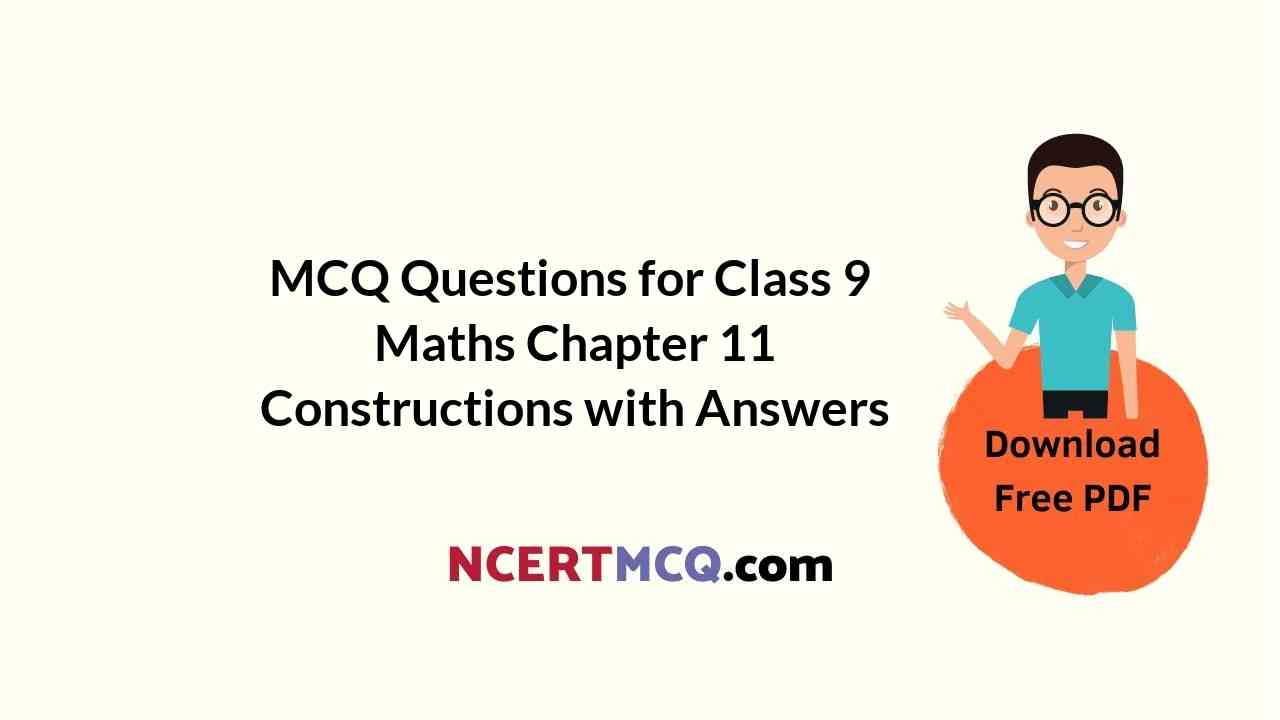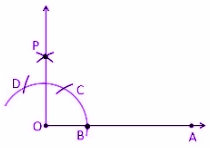Check the below Online Education NCERT MCQ Questions for Class 9 Maths Chapter 11 Constructions with Answers Pdf free download. MCQ Questions for Class 9 Maths with Answers were prepared based on the latest exam pattern. We have provided Constructions Class 10 Maths MCQs Questions with Answers to help students understand the concept very well. https://ncertmcq.com/mcq-questions-for-class-9-maths-with-answers/

Students can also refer to NCERT Solutions for Class 9 Maths Chapter 11 Constructions for better exam preparation and score more marks.

## Online Education for Constructions Class 9 MCQs Questions with Answers

Construction Class 9 MCQ Chapter 11 Question 1.
If a, b and c are the lengths of the three sides of a triangle, then which of the following is true?
(a) a + b < c
(b) a – b < c
(c) a + b = c

Answer: (b) a – b < c

Construction MCQ Class 9 Chapter 11 Question 2.
With the help of a ruler and compasses, which of the following is not possible to construct?
(a) 70°
(b) 60°
(c) 135°

Class 9 Construction MCQ Chapter 11 Question 3.
Which of the following sets of angles can be the angles of a triangle?
(a) 30°, 60°, 80°
(b) 40°, 60°, 70°
(c) 50°, 30°, 100°

Class 9 Maths Chapter 11 MCQ Question 4.
The construction of the triangle ABC is possible if it is given that BC = 4 cm, ∠C = 60° and the difference of AB and AC is
(a) 3.5 cm
(b) 4.5 cm
(c) 3 cm
(d) 2.5 cm

Class 9 Maths Ch 11 MCQ Question 5.
Which of the following can be the length of BC required to construct the triangle ABC such that AC = 7.4 cm and AB = 5 cm?
(a) 3.5 cm
(b) 2.1 cm
(c) 4.7 cm

Constructions Class 9 MCQ Chapter 11 Question 6.
If we want to construct a triangle, given its perimeter, then we need to know:
(a) Sum of two sides of triangle
(b) Difference between two sides of triangle
(c) One base angles
(d) Two base angles

MCQ On Constructions Class 9 Chapter 11 Question 7.
To construct a bisector of a given angle, we need:
(a) A ruler
(b) A compass
(c) A protractor
(d) Both ruler and compass

Answer: (d) Both ruler and compass

Class 9 Maths Chapter 11 MCQ With Answers Question 8.
Which of the following set of lengths can be the sides of a triangle?
(a) 2 cm, 4 cm, 1.9 cm
(b) 1.6 cm, 3.7 cm, 5.3 cm
(c) 5.5 cm, 6.5 cm, 8.9 cm
(d) None of the above

Answer: (c) 5.5 cm, 6.5 cm, 8.9 cm

MCQ Of Construction Class 9 Chapter 11 Question 9.
Which of these angles cannot be constructed using ruler and compasses?
(a) 120
(b) 60
(c) 140
(d) 135

MCQ Questions For Class 9 Maths Ncert Chapter 11 Question 10.
Which of the following angles can be constructed using ruler and compasses?
(a) 35
(b) 45
(c) 95
(d) 55

Hint:
See the figure below:ROA = 45°

Construction Class 9 MCQ With Answers Chapter 11 Question 11.
With the help of a ruler and compasses which of the following is not possible to construct?
(a) 120°
(b) 135°
(c) 140°

Class 9 Chapter 11 MCQ Question 12.
If the construction of a triangle ABC in which AB = 6 cm, ∠A = 70° and ∠B = 40° is possible then find the measure of ∠c.
(a) 40°
(b) 70°
(c) 80°

MCQ Questions For Class 9 Maths Chapter 11 Question 13.
Which of the following set of lengths can be the sides of a triangle?
(a) 2 cm, 4 cm, 1.9 cm
(b) 5.5 cm, 6.5 cm, 8.9 cm
(c) 1.6 cm, 3.7 cm. 5.3 cm

Answer: (b) 5.5 cm, 6.5 cm, 8.9 cm

Question 14.
The construction of a triangle ΔABC in which BC = 6 cm, ∠A = 50° is not possible, when difference of BC and AC is equal to
(a) 4.6 cm
(b) 6.4 cm
(c) 5.1 cm

Question 15.
Which of the following angle can be constructed with the help of a ruler and a pair of compasses?
(a) 35°
(b) 40°
(c) 37.5°
(d) 47.5°

Question 16.
To construct an angle of 60 degrees, we need to draw first:
(a) A ray
(b) An arc
(c) Two rays
(d) A straight line

Hint:
To construct an angle of 60 degrees we need to start at the initial point of a ray.

Question 17.
The side lengths 4 cm, 4 cm and 4 cm can be sides of:
(a) Scalene Triangle
(b) Isosceles Triangle
(c) Equilateral Triangle
(d) None of the above

Hint:
Equilateral triangle has all its three sides equal.

Question 18.
If a, b and c are the lengths of three sides of a triangle, then:
(a) a + b > c
(b) a – b > c
(c) a + b = c
(d) a – b = c

Answer: (a) a + b > c
Hint:
The sum of two sides of a triangle is always greater than the third side.

Question 19.
Which of these angles we cannot construct it using ruler and compasses?
(a) 120
(b) 70
(c) 60
(d) All can be constructed

Question 20
Which of the following angles can be constructed using ruler and compass?
(a) 35
(b) 40
(c) 90
(d) 50